maths > construction-basics

Constructing 60${60}^{\circ}$ angle using Compass

what you'll learn...

overview

• 60${60}^{\circ}$ angle

• 30${30}^{\circ}$ angle

• 120${120}^{\circ}$ angle

• 15${15}^{\circ}$ angle

• 90${90}^{\circ}$ angle

Constructing ${60}^{\circ}$ angle using Compass

We know that, Angles in a equilateral triangle are ${60}^{\circ}$. This geometrical property helps to construct ${60}^{\circ}$ angle using a compass.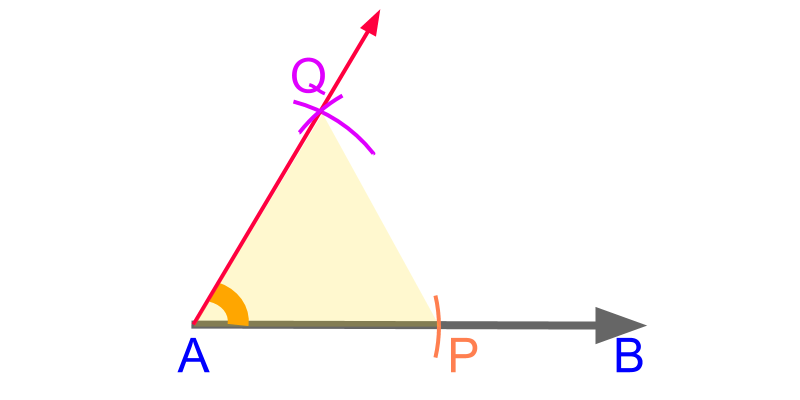To construct an angle measuring ${60}^{\circ}$ at point $A$ on line $\overline{A B}$, construct a equilateral triangle as given below.

•  With an arbitrary distance measure on compass, mark point $P$ from point $A$ on line $\overline{A B}$.

•  With the same distance measure on compass, construct two arcs from points $A$ and $P$. The arcs cut at point $Q$.

Since the distance measure on compass is identical, points $A$, $P$, and $Q$ make an equilateral triangle. The line $\overline{A Q}$ is extended and $\angle P A Q$ is ${60}^{\circ}$

Constructing ${60}^{\circ}$ angle using a Compass : Construct an equilateral triangle and the angle ${60}^{\circ}$ is constructed at the vertex.

Constructing ${30}^{\circ}$ angle using Compass

To construct ${30}^{\circ}$ angle using a compass, we use the property that ${30}^{\circ}$ is half of ${60}^{\circ}$.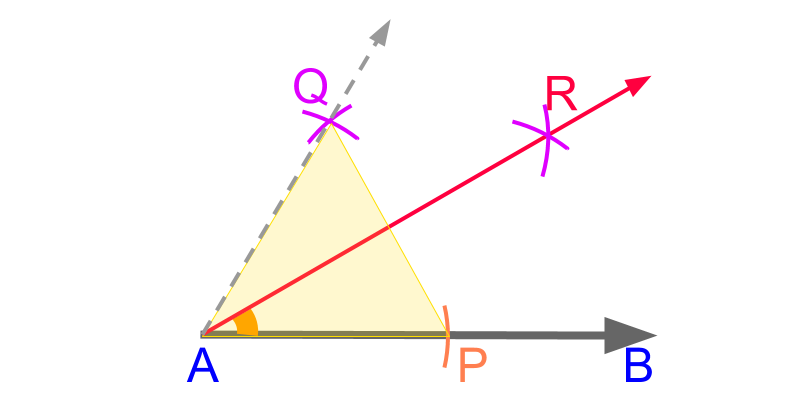We have learned constructing ${60}^{\circ}$ and bisecting an angle using a compass. Combine these two to construct ${30}^{\circ}$ angle.

•  With an arbitrary distance-measure on compass, mark point $P$ from point $A$ on line $\overline{A B}$.

•  With the same distance measure on compass, construct two arcs from points $A$ and $P$. The arcs cut at point $Q$. $\overline{A Q}$ makes ${60}^{\circ}$ with the $\overline{A P}$.

•  With an arbitrary distance-measure on compass, construct two arcs from points $P$ and $Q$. These arcs cut at point $R$. The line $\overline{A R}$ bisects the angle $\angle P A Q$.

The angle ${30}^{\circ}$ is constructed as $\angle P A R$.

Constructing ${30}^{\circ}$ angle using a Compass : Construct an equilateral triangle and the angle ${60}^{\circ}$ is constructed at the vertex. Bisect the angle.

Constructing ${120}^{\circ}$ angle using Compass

To construct ${120}^{\circ}$ angle using a compass, we use the property that ${120}^{\circ}$ is double of ${60}^{\circ}$.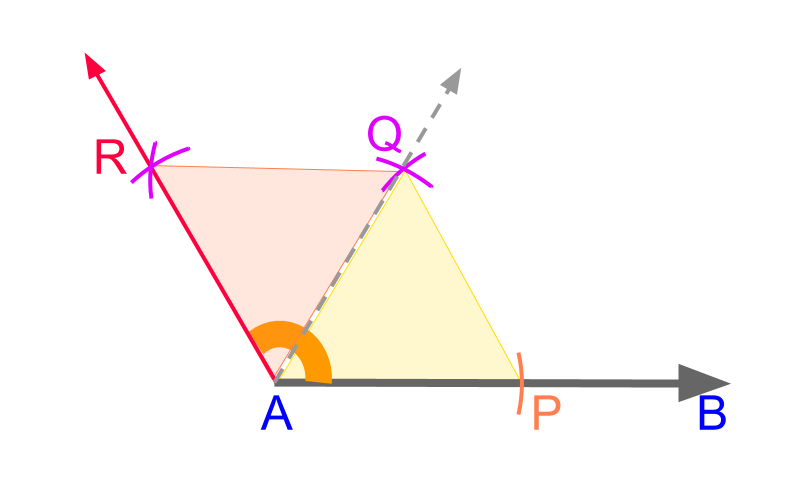Constructing ${60}^{\circ}$ angle twice-over to construct ${120}^{\circ}$

•  With an arbitrary distance-measure on compass, mark point $P$ from point $A$ on line $\overline{A B}$.

•  With the same distance measure on compass, construct two arcs from points $A$ and $P$. The arcs cut at point $Q$. $\overline{A Q}$ makes ${60}^{\circ}$ with the $\overline{A P}$.

•  With the same distance measure on compass, construct two arcs from points $A$ and $Q$. The arcs cut at point $R$. $\overline{A R}$ makes ${120}^{\circ}$ with the $\overline{A P}$. The angle ${120}^{\circ}$ is constructed as $\angle P A R$.

Constructing ${120}^{\circ}$ angle using a Compass : Construct an equilateral triangle and another equilateral triangle on the side of the constructed one.

Constructing ${15}^{\circ}$ angle using Compass

To construct ${15}^{\circ}$ angle using a compass, we use the property that ${15}^{\circ}$ is half of ${30}^{\circ}$.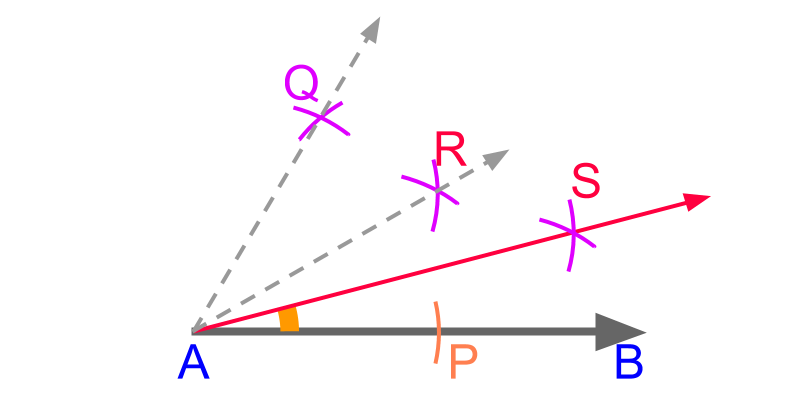We have learned constructing ${60}^{\circ}$ and bisecting an angle using a compass. Combine these two to construct ${15}^{\circ}$ angle.

•  With an arbitrary distance-measure on compass, mark point $P$ from point $A$ on line $\overline{A B}$.

•  With the same distance measure on compass, construct two arcs from points $A$ and $P$. The arcs cut at point $Q$. $\overline{A Q}$ makes ${60}^{\circ}$ with the $\overline{A P}$.

•  With an arbitrary distance-measure on compass, construct two arcs from points $P$ and $Q$. These arcs cut at point $R$. The line $\overline{A R}$ bisects the angle $\angle P A Q$.

•  With an arbitrary distance-measure on compass, construct two arcs from points $P$ and $R$. These arcs cut at point $S$. The line $\overline{A S}$ bisects the angle $\angle P A S$.

The angle ${15}^{\circ}$ is constructed as $\angle P A S$.

Constructing ${15}^{\circ}$ angle using a Compass : Construct an equilateral triangle and the angle ${60}^{\circ}$ is constructed at the vertex. Bisect the angle twice.

Constructing ${90}^{\circ}$ angle using Compass

To construct ${90}^{\circ}$ angle using a compass, we use the property that ${90}^{\circ}$ is sum of ${60}^{\circ}$ and ${30}^{\circ}$.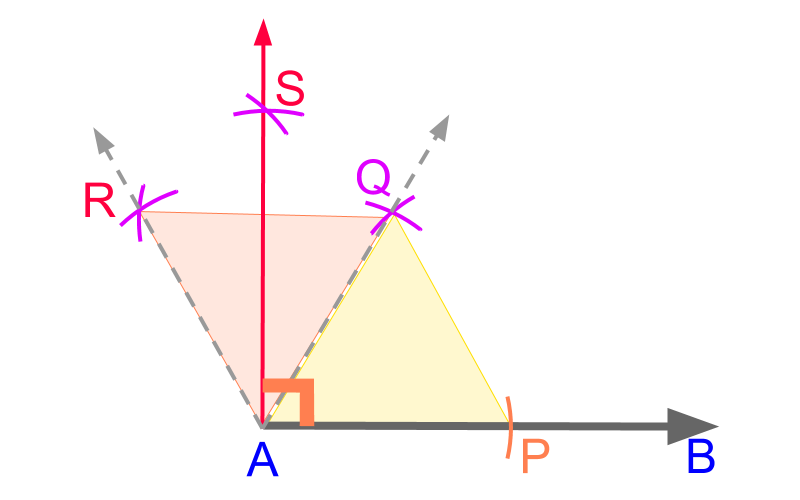Constructing ${90}^{\circ}$ with two ${60}^{\circ}$ is illustrated

•  With an arbitrary distance-measure on compass, mark point $P$ from point $A$ on line $\overline{A B}$.

•  With the same distance measure on compass, construct two arcs from points $A$ and $P$. The arcs cut at point $Q$. $\overline{A Q}$ makes ${60}^{\circ}$ with the $\overline{A P}$.

•  With the same distance measure on compass, construct two arcs from points $A$ and $Q$. The arcs cut at point $R$. $\overline{A R}$ makes ${120}^{\circ}$ with the $\overline{A P}$.

•  With an arbitrary distance-measure on compass, construct two arcs from points $Q$ and $R$. These arcs cut at point $S$. The line $\overline{A S}$ bisects the angle $\angle Q A R$.

The angle ${90}^{\circ}$ is constructed as $\angle P A S$.

Constructing ${90}^{\circ}$ angle using a compass : Construct two equilateral triangles and bisect the second one to construct ${90}^{\circ}$ angle.

summary

Constructing ${60}^{\circ}$ angle using a Compass : Construct an equilateral triangle and the angle ${60}^{\circ}$ is constructed at the vertex.

Constructing ${30}^{\circ}$ angle using a Compass : Construct an equilateral triangle and the angle ${60}^{\circ}$ is constructed at the vertex. Bisect the angle.

Constructing ${120}^{\circ}$ angle using a Compass : Construct an equilateral triangle and another equilateral triangle on the side of the constructed one.

Constructing ${15}^{\circ}$ angle using a Compass : Construct an equilateral triangle and the angle ${60}^{\circ}$ is constructed at the vertex. Bisect the angle twice.

Constructing ${90}^{\circ}$ angle using a compass : Construct two equilateral triangles and bisect the second one to construct ${90}^{\circ}$ angle.

Outline